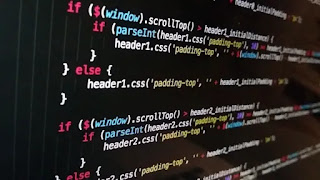# Python String Contains substring - Length of string

Yes the python strings contains substring and length of string is  not fix by python programmer developer. In this section we learn about the python string, python string contains and Explain the python length. First we learn about the python sting explain, String method, string length and string contains substring.Python string contains substring

## Python string

python string are surrounded by double and single quotation marks. Python String is the keyword of data type. Example of data types keyword are int, strings, float, boolen, decimal, float. This data type keyword is use for store data in variable. Multiline strings is create by using third quotation marks.

Example of string

``a = "codebugfree" ``

Example of multiline string

``````a = ''' Codebugfree is a awesome website to learn  the code and programming
We learn about Does python string contains substring.
What is the maximum length of string in python programming'''``````

Now we learn Python string function. The most common function to use in python are startwith,endswith, len, reverse and sort, Now we see the example of python string fuction

``````story = "hello my name is code free and i am a good boy and i can do bath every morning"
#string fuction
print(len(story))
print(story.endswith("morning"))
print(story.count("a"))
print(story.capitalize())
print(story.find("code"))
print(story.replace("code", "hanucharya"))``````

We first make the string and save the strings in story variable. len is the find the total length of string. endswith is the return boolen valuses means true and false. count is the counting the specific value which you want. capitalize is change your first alphabet in capital letters. find is the finding that value or alphabet which you want. replace is use to replace your old word into new word.

INOUTPUT

``````78
True
7
Hello my name is code free and i am a good boy and i can do bath every morning
17
hello my name is hanucharya free and i am a good boy and i can do bath every morning``````

### Python contains substring

``````user_input = input("Enter a your string: ")
#substring
#codebugfree
for i in range(len(user_input)):
for j in range(i+1,len(user_input)+1):
#len length of string
print(user_input[i:j])``````

How to write this program. Getting from user input as string. Starting a for loop with range len mans length of user input string again making for loop inside the for loop range start from i+1 and end user input string length +1. print user input as a string slicing

INOUTPUT

``````Enter a your string: python
p
py
pyt
pyth
pytho
python
y
yt
yth
ytho
ython
t
th
tho
thon
h
ho
hon
o
on
n``````

#### Python string length length

Any string find the total length of string sentences or word use the len() function. It is a built in python function. Helps of len() function calculated the length. See  the example

Example

``````Python_programmer = input("Enter your word: ")
print(len(Python_programmer))``````

First string value input from user and user input value length is caculated by len function

``````Enter your word: i am a python programmer. Learn from internet
45``````